# Chapter 6 Hypothesis tests Hypothesis tests Statistical tests

• Slides: 18Chapter 6 Hypothesis tests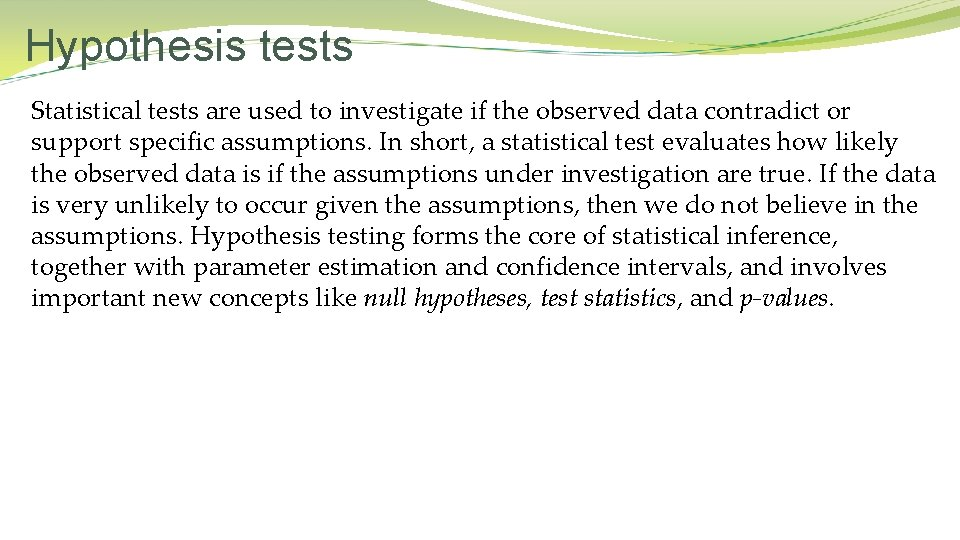Hypothesis tests Statistical tests are used to investigate if the observed data contradict or support specific assumptions. In short, a statistical test evaluates how likely the observed data is if the assumptions under investigation are true. If the data is very unlikely to occur given the assumptions, then we do not believe in the assumptions. Hypothesis testing forms the core of statistical inference, together with parameter estimation and confidence intervals, and involves important new concepts like null hypotheses, test statistics, and p-values.Concepts of hypothesis test Null hypothesis. A null hypothesis is a simplification of the statistical model and is as such always related to the statistical model. Hence, no null hypothesis exists without a corresponding statistical model. A null hypothesis typically describes the situation of “no effect” or “no relationship”, such that rejection of the null hypothesis corresponds to evidence of an effect or relationship. Alternative hypothesis. There is a corresponding alternative hypothesis to every null hypothesis. The alternative hypothesis describes what is true if the null hypothesis is false. Usually the alternative hypothesis is simply the complement of the null hypothesis.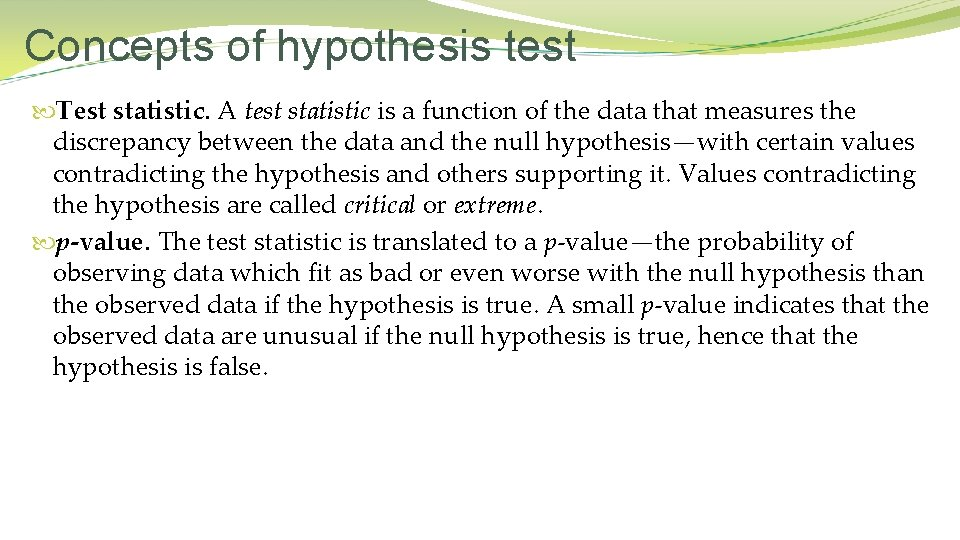Concepts of hypothesis test Test statistic. A test statistic is a function of the data that measures the discrepancy between the data and the null hypothesis—with certain values contradicting the hypothesis and others supporting it. Values contradicting the hypothesis are called critical or extreme. p-value. The test statistic is translated to a p-value—the probability of observing data which fit as bad or even worse with the null hypothesis than the observed data if the hypothesis is true. A small p-value indicates that the observed data are unusual if the null hypothesis is true, hence that the hypothesis is false.Concepts of hypothesis test Rejection. The hypothesis is rejected if the p-value is small; namely, below (or equal to) the significance level, which is often taken to be 0. 05. With statistics we can at best reject the null hypothesis with strong certainty, but we can never confirm the hypothesis. If we fail to reject the null hypothesis, then the only valid conclusion is that the data do not contradict the null hypothesis. A large p-value shows that the data are in fine accordance with the null hypothesis, but not that it is true. Quantification of effects. Having established a significant effect by a hypothesis test, it is of great importance to quantify the effect. For example, how much larger is the expected hormone concentration after a period of treatment? Moreover, what is the precision of the estimates in terms of standard errors and/or confidence intervals?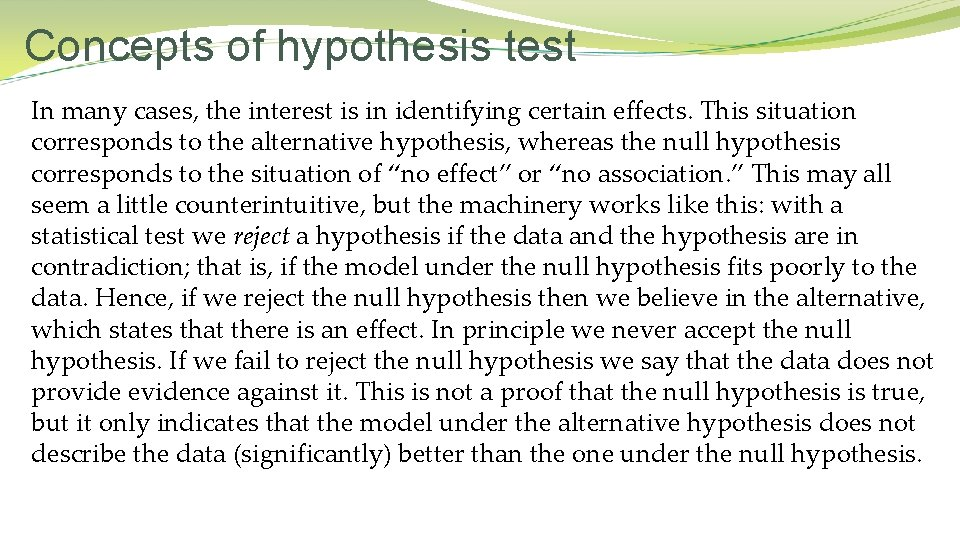Concepts of hypothesis test In many cases, the interest is in identifying certain effects. This situation corresponds to the alternative hypothesis, whereas the null hypothesis corresponds to the situation of “no effect” or “no association. ” This may all seem a little counterintuitive, but the machinery works like this: with a statistical test we reject a hypothesis if the data and the hypothesis are in contradiction; that is, if the model under the null hypothesis fits poorly to the data. Hence, if we reject the null hypothesis then we believe in the alternative, which states that there is an effect. In principle we never accept the null hypothesis. If we fail to reject the null hypothesis we say that the data does not provide evidence against it. This is not a proof that the null hypothesis is true, but it only indicates that the model under the alternative hypothesis does not describe the data (significantly) better than the one under the null hypothesis.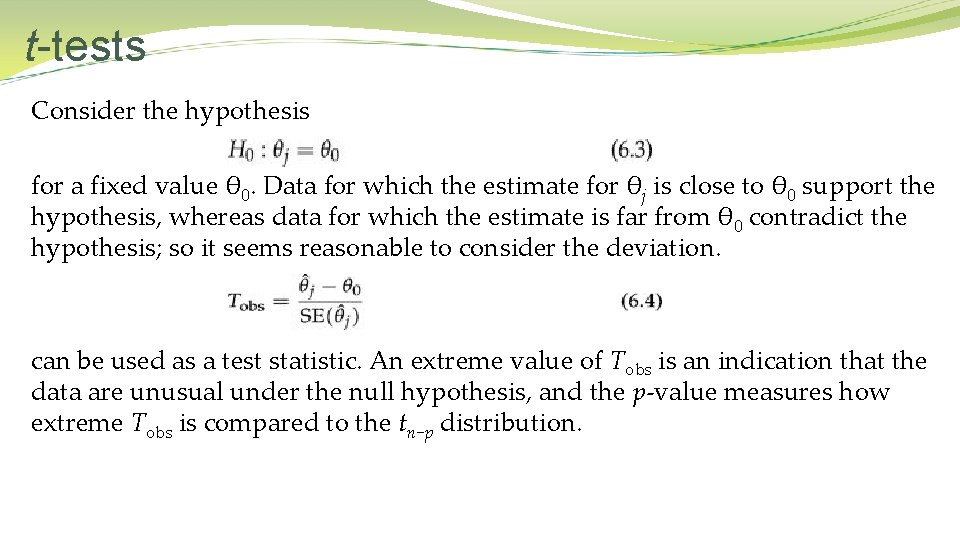t-tests Consider the hypothesis for a fixed value θ 0. Data for which the estimate for θj is close to θ 0 support the hypothesis, whereas data for which the estimate is far from θ 0 contradict the hypothesis; so it seems reasonable to consider the deviation. can be used as a test statistic. An extreme value of Tobs is an indication that the data are unusual under the null hypothesis, and the p-value measures how extreme Tobs is compared to the tn−p distribution.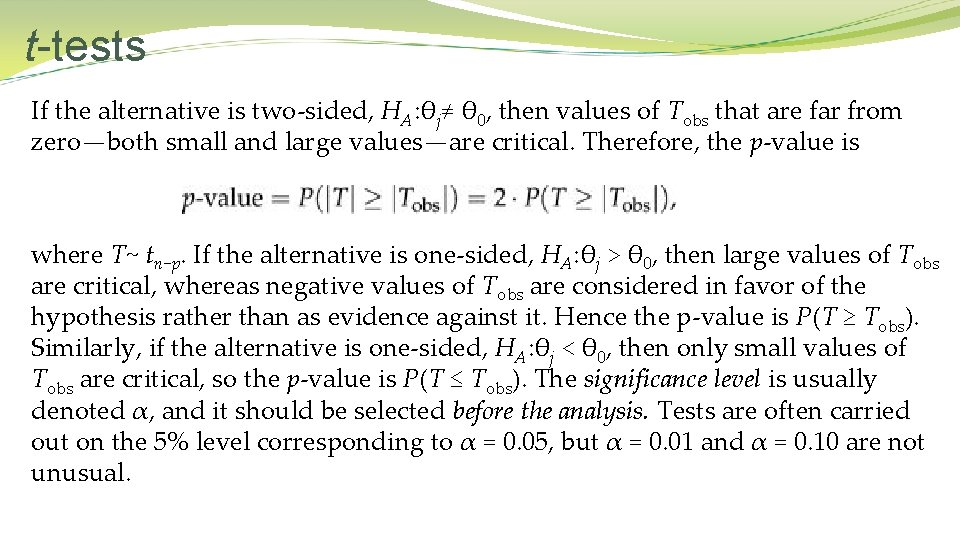t-tests If the alternative is two-sided, HA: θj≠ θ 0, then values of Tobs that are far from zero—both small and large values—are critical. Therefore, the p-value is where T~ tn−p. If the alternative is one-sided, HA: θj > θ 0, then large values of Tobs are critical, whereas negative values of Tobs are considered in favor of the hypothesis rather than as evidence against it. Hence the p-value is P(T ≥ Tobs). Similarly, if the alternative is one-sided, HA: θj < θ 0, then only small values of Tobs are critical, so the p-value is P(T ≤ Tobs). The significance level is usually denoted α, and it should be selected before the analysis. Tests are often carried out on the 5% level corresponding to α = 0. 05, but α = 0. 01 and α = 0. 10 are not unusual.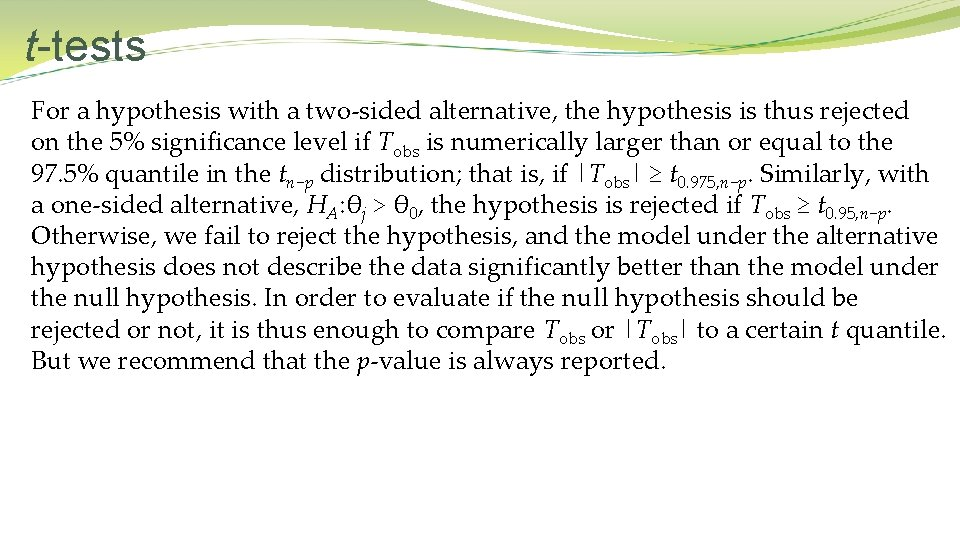t-tests For a hypothesis with a two-sided alternative, the hypothesis is thus rejected on the 5% significance level if Tobs is numerically larger than or equal to the 97. 5% quantile in the tn−p distribution; that is, if |Tobs| ≥ t 0. 975, n−p. Similarly, with a one-sided alternative, HA: θj > θ 0, the hypothesis is rejected if Tobs ≥ t 0. 95, n−p. Otherwise, we fail to reject the hypothesis, and the model under the alternative hypothesis does not describe the data significantly better than the model under the null hypothesis. In order to evaluate if the null hypothesis should be rejected or not, it is thus enough to compare Tobs or |Tobs| to a certain t quantile. But we recommend that the p-value is always reported.t-tests and confidence intervals H 0: θj = θ 0 is rejected on significance level α against the alternative HA: θj≠θ 0 if and only if θ 0 is not included in the 1−α confidence interval. This relationship explains the formulation about confidence intervals; namely, that a confidence interval includes the values that are in accordance with the data. This now has a precise meaning in terms of hypothesis tests. If the only aim of the analysis is to conclude whether a hypothesis should be rejected or not at a certain level α, then we get that information from either the t-test or the confidence interval. On the other hand, they provide extra information on slightly different matters. The t-test provides a p-value explaining how extreme the observed data are if the hypothesis is true, whereas the confidence interval gives us the values of θ that are in agreement with the data.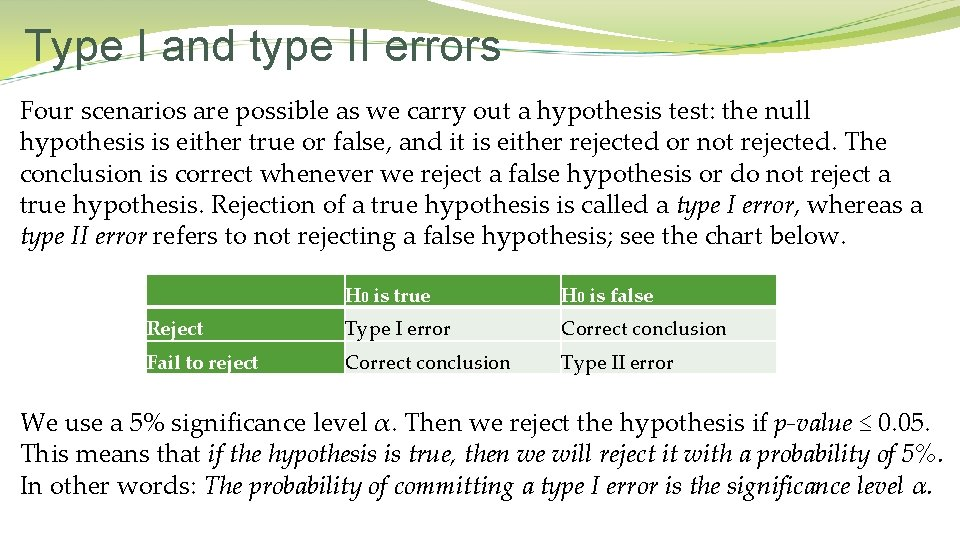Type I and type II errors Four scenarios are possible as we carry out a hypothesis test: the null hypothesis is either true or false, and it is either rejected or not rejected. The conclusion is correct whenever we reject a false hypothesis or do not reject a true hypothesis. Rejection of a true hypothesis is called a type I error, whereas a type II error refers to not rejecting a false hypothesis; see the chart below. H 0 is true H 0 is false Reject Type I error Correct conclusion Fail to reject Correct conclusion Type II error We use a 5% significance level α. Then we reject the hypothesis if p-value ≤ 0. 05. This means that if the hypothesis is true, then we will reject it with a probability of 5%. In other words: The probability of committing a type I error is the significance level α.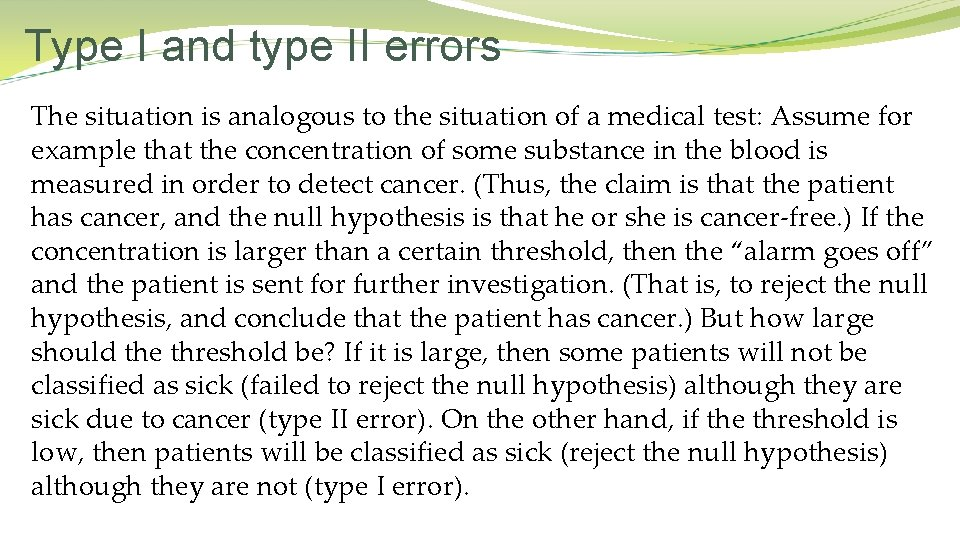Type I and type II errors The situation is analogous to the situation of a medical test: Assume for example that the concentration of some substance in the blood is measured in order to detect cancer. (Thus, the claim is that the patient has cancer, and the null hypothesis is that he or she is cancer-free. ) If the concentration is larger than a certain threshold, then the “alarm goes off” and the patient is sent for further investigation. (That is, to reject the null hypothesis, and conclude that the patient has cancer. ) But how large should the threshold be? If it is large, then some patients will not be classified as sick (failed to reject the null hypothesis) although they are sick due to cancer (type II error). On the other hand, if the threshold is low, then patients will be classified as sick (reject the null hypothesis) although they are not (type I error).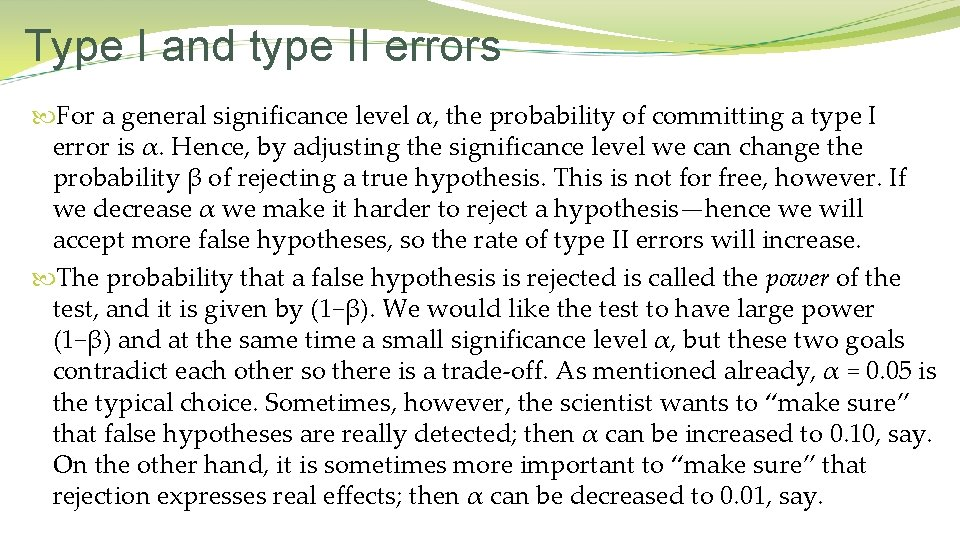Type I and type II errors For a general significance level α, the probability of committing a type I error is α. Hence, by adjusting the significance level we can change the probability β of rejecting a true hypothesis. This is not for free, however. If we decrease α we make it harder to reject a hypothesis—hence we will accept more false hypotheses, so the rate of type II errors will increase. The probability that a false hypothesis is rejected is called the power of the test, and it is given by (1−β). We would like the test to have large power (1−β) and at the same time a small significance level α, but these two goals contradict each other so there is a trade-off. As mentioned already, α = 0. 05 is the typical choice. Sometimes, however, the scientist wants to “make sure” that false hypotheses are really detected; then α can be increased to 0. 10, say. On the other hand, it is sometimes more important to “make sure” that rejection expresses real effects; then α can be decreased to 0. 01, say.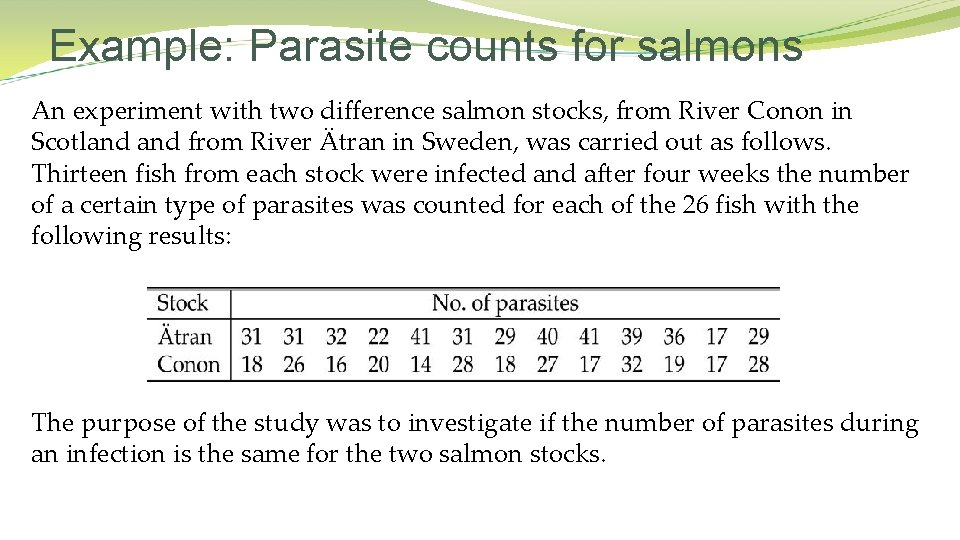Example: Parasite counts for salmons An experiment with two difference salmon stocks, from River Conon in Scotland from River Ätran in Sweden, was carried out as follows. Thirteen fish from each stock were infected and after four weeks the number of a certain type of parasites was counted for each of the 26 fish with the following results: The purpose of the study was to investigate if the number of parasites during an infection is the same for the two salmon stocks.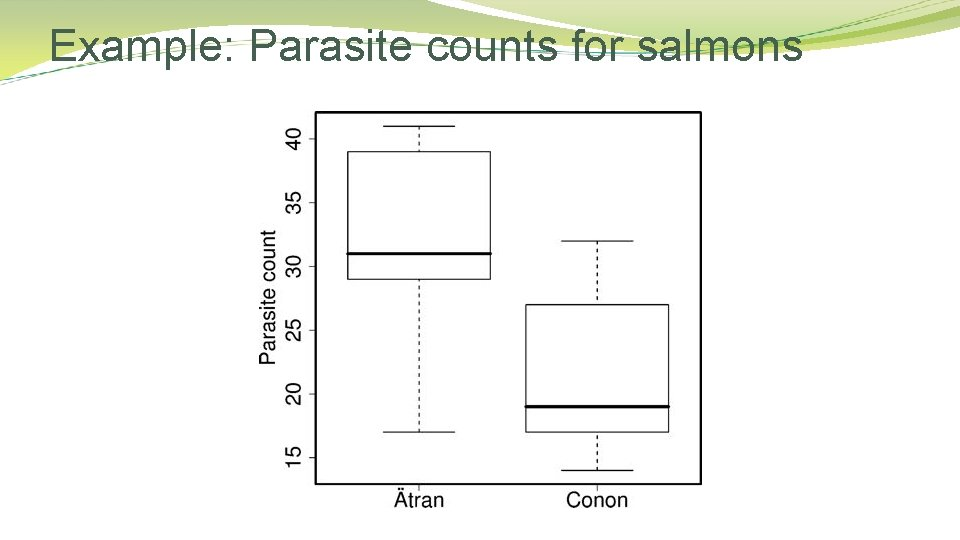Example: Parasite counts for salmonsExample: Parasite counts for salmons The mean and sample standard deviations are computed to The summary statistics and the boxplots tell the same story: The observed parasite counts are generally higher for the Ätran group compared to the Conon group, indicating that Ätran salmons are more susceptible to parasites. The purpose of the statistical analysis is to clarify whether the observed difference is caused by an actual difference between the stocks or by random variation.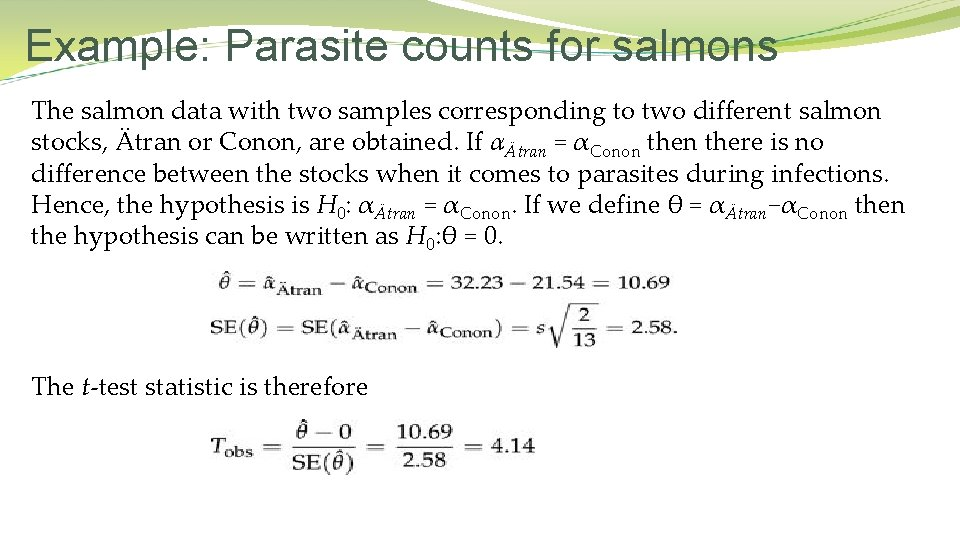Example: Parasite counts for salmons The salmon data with two samples corresponding to two different salmon stocks, Ätran or Conon, are obtained. If αÄtran = αConon there is no difference between the stocks when it comes to parasites during infections. Hence, the hypothesis is H 0: αÄtran = αConon. If we define θ = αÄtran−αConon the hypothesis can be written as H 0: θ = 0. The t-test statistic is therefore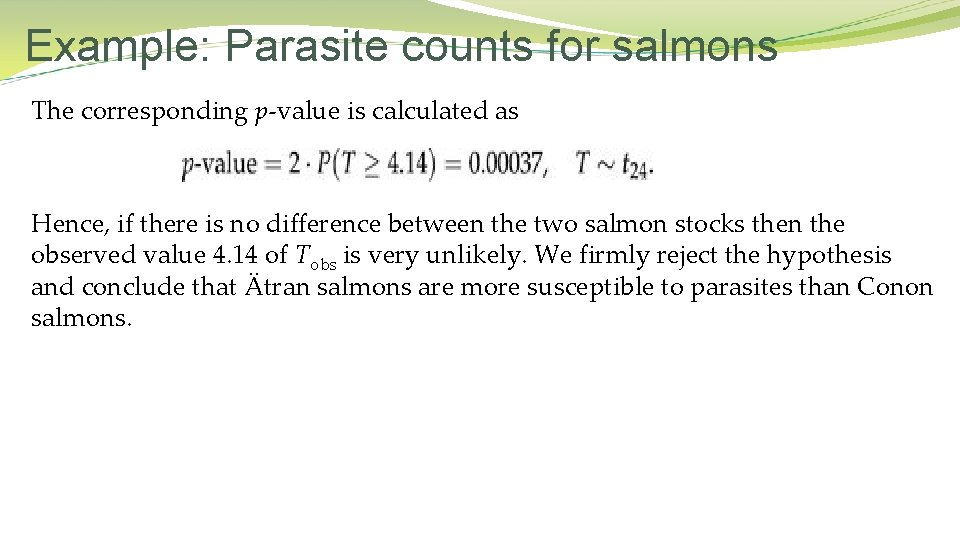Example: Parasite counts for salmons The corresponding p-value is calculated as Hence, if there is no difference between the two salmon stocks then the observed value 4. 14 of Tobs is very unlikely. We firmly reject the hypothesis and conclude that Ätran salmons are more susceptible to parasites than Conon salmons.